# Create and Explore Datastore for Image Classification

This example shows how to create, read, and augment an image datastore for use in training a deep learning network. In particular, this example shows how to create an `ImageDatastore` object from a collection of images, read and extract the properties of the datastore, and create an `augmentedImageDatastore` for use during training.

### Create Image Datastore

Use an `imageDatastore` object to manage a large collection of images that cannot altogether fit in memory. Large collections of images are common in deep learning applications, which regularly involve training on thousands of labeled images. These images are often stored in a folder, with subfolders containing images for each class.This example uses the Example Food Images data set, which contains 978 photographs of food in nine classes and is approximately 77 MB in size. Download the `ExampleFoodImageDataset.zip` file from the MathWorks website, then unzip the file.

```zipFile = matlab.internal.examples.downloadSupportFile('nnet','data/ExampleFoodImageDataset.zip'); filepath = fileparts(zipFile); dataFolder = fullfile(filepath,'ExampleFoodImageDataset'); unzip(zipFile,dataFolder); ```

The images in this data set are separated into subfolders for each class.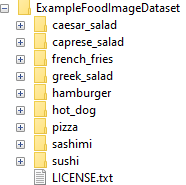Create an image datastore from the images in the path and their subfolders. Use the folder names as label names.

```foodImds = imageDatastore(dataFolder, ... 'IncludeSubfolders',true, ... 'LabelSource','foldernames');```

### Properties of Datastore

Extract the properties of the datastore.

Find the total number of observations. This data set has 978 observations split into nine classes.

`numObs = length(foodImds.Labels)`
```numObs = 978 ```

Find the number of observations per class. You can see that this data set does not contain an equal number of observations in each class.

`numObsPerClass = countEachLabel(foodImds)`
```numObsPerClass=9×2 table Label Count _____________ _____ caesar_salad 26 caprese_salad 15 french_fries 181 greek_salad 24 hamburger 238 hot_dog 31 pizza 299 sashimi 40 sushi 124 ```

You can also visualize the distribution of the class labels using a histogram.

```histogram(foodImds.Labels) set(gca,'TickLabelInterpreter','none')```### Explore Datastore

Check that the data is as expected by viewing a random selection of images from the datastore.

```numObsToShow = 8; idx = randperm(numObs,numObsToShow); imshow(imtile(foodImds.Files(idx),'GridSize',[2 4],'ThumbnailSize',[100 100]))```You can also view images that belong to a specific class.

```class =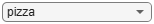"pizza"; idxClass = find(foodImds.Labels == class); idx = randsample(idxClass,numObsToShow); imshow(imtile(foodImds.Files(idx),'GridSize',[2 4],'ThumbnailSize',[100 100]));```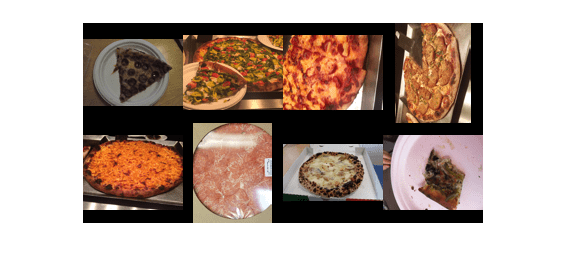To take a closer look at individual images in your datastore or folder, use the Image Browser (Image Processing Toolbox) app.

### Image Augmentation

Augmentation enables you to train networks to be invariant to distortions in image data. For example, you can add randomized rotations to input images so that a network is invariant to the presence of rotation. An `augmentedImageDatastore` object provides a convenient way to apply a limited set of augmentations to 2-D images for classification problems.

Define an augmentation scheme. This scheme applies a random rotation between [–90,90] degrees and a random scaling between [1,2]. The augmented datastore automatically resizes the images to the `inputSize` value during training.

```imageAugmenter = imageDataAugmenter( ... 'RandRotation',[-90 90], ... 'RandScale',[1 2]); inputSize = [100 100]; ```

Using the augmentation scheme, define the augmented image datastore.

```augFoodImds = augmentedImageDatastore(inputSize,foodImds, ... 'DataAugmentation',imageAugmenter);```

The augmented datastore contains the same number of images as the original image datastore.

`augFoodImds.NumObservations`
```ans = 978 ```

When you use an augmented image datastore as a source of training images, the datastore randomly perturbs the training data for each epoch, where an epoch is a full pass of the training algorithm over the entire training data set. Therefore, each epoch uses a slightly different data set, but the actual number of training images in each epoch does not change.

#### Visualize Augmented Data

Visualize the augmented image data that you want to use to train the network.

Shuffle the datastore.

`augFoodImds = shuffle(augFoodImds);`

The `augmentedImageDatastore` object applies the transformations when reading the datastore and does not store the transformed images in memory. Consequently, each time you read the same images, you see a random combination of the augmentations defined.

Use the `read` function to read a subset of the augmented datastore.

`subset1 = read(augFoodImds);`

Reset the datastore to its state before calling read and read a subset of the datastore again.

```reset(augFoodImds) subset2 = read(augFoodImds);```

Display the two subsets of the augmented images.

`imshow(imtile(subset1.input,'GridSize',[2 4]))`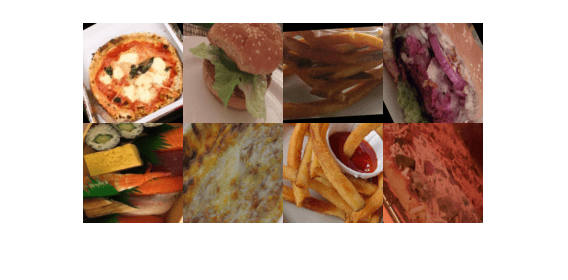`imshow(imtile(subset2.input,'GridSize',[2 4]))`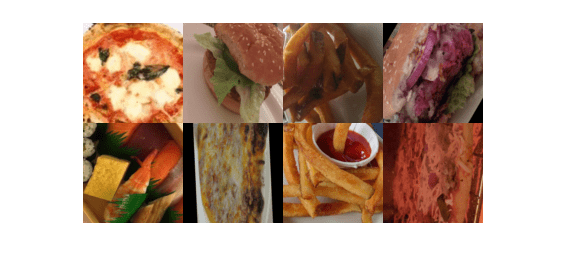You can see that both instances show the same images with different transformations. Applying transformations to images is useful in deep learning applications, as you can train the network on randomly altered versions of an image. Doing so exposes the network to different variations of images from that class and enables it to learn to classify images even if they have different visual properties.

After creating your datastore object, use the Deep Network Designer app or `trainNetwork` function to train an image classification network. For an example, see Transfer Learning Using Pretrained Network.

For more information on preprocessing images for deep learning applications, see Preprocess Images for Deep Learning. You can also apply more advanced augmentations, such as varying levels of brightness or saturation, by using the `transform` and `combine` functions. For more information, see Datastores for Deep Learning.